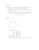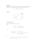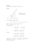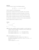# Exercises D pdfLoading previews...vector geometry in 2 dimensionsvector geometry in 2 dimensionsPappus' theorem using vectorssimultaneous equationsequations of planes
23 files in this resource

## Exercises D pdf

Exercises and solutions in PDFView Item